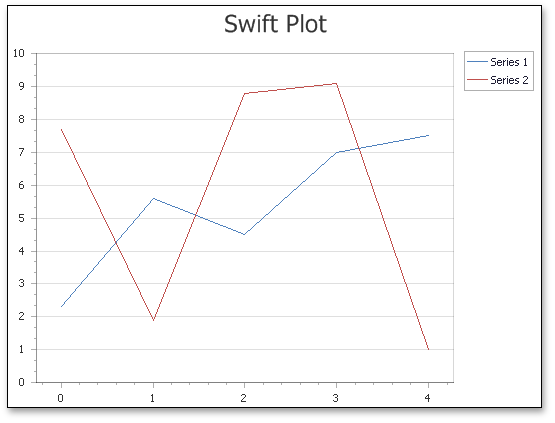21.1 (EAP/Beta)
20.2
20.1
19.2
19.1
18.2
18.1
17.2

# Swift Plot

## Short Description

The Swift Plot is represented by the SwiftPlotSeriesView object, which belongs to Point, Line and Spline Series Views.

This view is intended for quick series rendering, based on a lightened charts generation algorithm. This view is primarily used when it’s required either to display a large amount of data points (tens of thousands and more), and/or create a real-time chart that reflects processes and is updated (by adding new points) in short time spans (e.g. milliseconds). Thanks to the specially introduced algorithm, series of this view type easily manage such tasks with much better performance than any other view.Note that to provide the best possible performance, the Swift Plot lacks some elements and features available for other view types. These features are listed below.

However, the most important capabilities are still available for the Swift Plot (e.g. panes, secondary axes, financial indicators and regression lines, and the WebChartControl.CustomDrawSeries event).

## Chart Type Characteristics

Although the Swift Plot belongs to Point and Line series, it is incompatible with other views of this group, because it uses a special SwiftPlotDiagram type. So, within a single chart control you can only display Swift Plot series, while this limitation doesn’t restrict using multiple Swift Plot series within the same chart (either in a single, or separate panes).

##### NOTE

The Swift Plot is designed to process primarily numerical and date-time data. So, the declared performance isn’t guaranteed when the qualitative scale-type is specified for the series SeriesBase.ArgumentScaleType property. For more information, refer to Series Scale Types.

A notable option available for series of this type is the SwiftPlotSeriesView.Antialiasing property, which allows you to enable smooth series drawing.

The table below lists the main characteristics of this chart type.

Feature Value
Series View type SwiftPlotSeriesView
Diagram type SwiftPlotDiagram
Number of arguments per series point 1
Number of values per series point 1

## Example

This example demonstrates how to use the SwiftPlotDiagram to display a large data source without freezes.

``````public partial class _Default : Page {
protected void Page_Load(object sender, EventArgs e) {
Series swiftPlotSeries = new Series {
Name = "Swift Plot Series",
View = new SwiftPlotSeriesView()
};
PopulateSeriesWithData(swiftPlotSeries, 100000);

SwiftPlotDiagram diagram = chartControl.Diagram as SwiftPlotDiagram;
CustomizeSwiftPlotDiagram(diagram);
}

protected void CustomizeSwiftPlotDiagram(SwiftPlotDiagram diagram) {
if (diagram == null) return;
diagram.AxisX.Title.Visibility = DefaultBoolean.True;
diagram.AxisX.Title.Text = "Point Number";
diagram.AxisX.NumericScaleOptions.ScaleMode = ScaleMode.Manual;
diagram.AxisX.NumericScaleOptions.MeasureUnit = NumericMeasureUnit.Custom;
diagram.AxisX.NumericScaleOptions.CustomMeasureUnit = 1000;

diagram.AxisX.NumericScaleOptions.AggregateFunction = AggregateFunction.Average;

diagram.AxisY.Title.Visibility = DefaultBoolean.True;
diagram.AxisY.Title.Text = "Point Value";
}

protected void PopulateSeriesWithData(Series series, int dataPoints) {
Random rnd = new Random();
for (int i = 0; i < dataPoints; ++i) {
new SeriesPoint(
argument: i,
values: 2 * Math.Cos((double)i / dataPoints * 2 * Math.PI)
+ Math.Sin((double)i / dataPoints * 2 * Math.PI)
+ 4 * Math.Cos(2 * (double)i / dataPoints * 2 * Math.PI)
+ 5 * Math.Sin(2 * (double)i / dataPoints * 2 * Math.PI)
+ 0.6 * rnd.NextDouble() - 0.3
)
);
}
}
}
``````
##### TIP

A complete sample project is available in the DevExpress Code Examples database at http://www.devexpress.com/example=E1836.